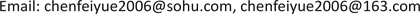﻿ 基于面板数据模型的险资投资效率影响因素实证研究 The Empirical Study on Factors Influencing Investment Efficiency of Insurance Funds Based on the Panel Data Model

Finance
Vol.07 No.05(2017), Article ID:22861,7 pages
10.12677/FIN.2017.75032

The Empirical Study on Factors Influencing Investment Efficiency of Insurance Funds Based on the Panel Data Model

Feiyue Chen

Department of Finance, Insurance Professional College, Changsha HunanReceived: Nov. 7th, 2017; accepted: Nov. 22nd, 2017; published: Nov. 29th, 2017ABSTRACT

Return on investment is the major source of profits of insurance companies. Investment efficiency of insurance funds reflects ability of capital operation of insurance companies, which is crucial of great importance to the continuous stable operation and long-term development of insurance companies. By using panel data of 23 insurance companies of China in 2007-2014 on an empirical study on relationship between investment efficiency of insurance funds and factors influencing investment efficiency, result shows that asset size is negatively correlated with using efficiency of insurance funds; fixed term deposit-rate has significantly negative effects on investment efficiency of insurance funds, long-term securities investment-rate does prominently positively; Market share significantly improves investment efficiency; Comprehensive operating fee-rate has a negative effect on investment efficiency. The quality of investment department staffs plays a positive role on investment efficiency, but results are not significant.

Keywords:Insurance Funds, Investment Efficiency, Panel Data1. 引言

2014年《国务院关于加快发展现代保险服务业的若干意见》明确指出，要充分发挥保险资金长期投资的独特优势，在保证安全性、收益性前提下，创新保险资金运用方式，提高保险资金配置效率。

2. 面板数据计量经济模型

2.1. 面板数据(Panel Data)模型概述

${y}_{it}={\alpha }_{it}+{{x}^{\prime }}_{it}{\beta }_{it}+{\mu }_{it},\text{\hspace{0.17em}}\text{\hspace{0.17em}}i=1,2,\cdots ,N;\text{\hspace{0.17em}}t=1,2,\cdots ,T$ (1)

Panel Data模型(1)可简化为如下形式：

${y}_{i}={\alpha }_{i}e+{x}_{i}{\beta }_{i}+{\mu }_{i},\text{\hspace{0.17em}}\text{\hspace{0.17em}}e={\left(1,1,\cdots ,1\right)}^{\prime },\text{\hspace{0.17em}}i=1,2,\cdots ,N$ (2)

1) 变系数模型：即截距不同，回归系数也不同

${y}_{i}={\alpha }_{i}e+{x}_{i}{\beta }_{i}+{\mu }_{i},\text{\hspace{0.17em}}\text{\hspace{0.17em}}i=1,2,\cdots ,N$

2) 变截距模型：即截距不同，回归系数相同

${y}_{i}={\alpha }_{i}e+{x}_{i}\beta +{\mu }_{i},\text{\hspace{0.17em}}\text{\hspace{0.17em}}i=1,2,\cdots ,N$

3) 混合模型：即截距相同，回归系数相同

${y}_{i}=\alpha e+{x}_{i}\beta +{\mu }_{i},\text{\hspace{0.17em}}\text{\hspace{0.17em}}i=1,2,\cdots ,N$

${H}_{1}:{\beta }_{1}={\beta }_{2}=\cdots ={\beta }_{N}$ , ${\alpha }_{i}$ 不完全相等(混合回归模型)

${H}_{2}:{\beta }_{1}={\beta }_{2}=\cdots ={\beta }_{N},\text{\hspace{0.17em}}{\alpha }_{1}={\alpha }_{2}=\cdots ={\alpha }_{N}$ (个体固定效应回归模型)

${S}_{1},{S}_{2},{S}_{3}$ 分别为变系数模型(i)、变截距模型(ii)和混合模型(iii)的残差平方和，则在假设 ${H}_{2}$ 成立的条件下，统计量 ${F}_{2}$ 服从F分布，即

${F}_{2}=\frac{\left({S}_{3}-{S}_{1}\right)/\left[\left(N-1\right)\left(k+1\right)\right]}{{S}_{1}/\left[NT-N\left(k+1\right)\right]}~F\left[\left(N-1\right)\left(k+1\right),N\left(T-k-1\right)\right]$

${F}_{1}=\frac{\left({S}_{2}-{S}_{1}\right)/\left[\left(N-1\right)\left(k+1\right)\right]}{{S}_{1}/\left[NT-N\left(k+1\right)\right]}~F\left[\left(N-1\right)k,N\left(T-k-1\right)\right]$

$W={\left[b-\stackrel{^}{\beta }\right]}^{\prime }{\varnothing }^{-1}\left[b-\stackrel{^}{\beta }\right]$

Hausman证明在原假设下，统计量 $W$ 服从自由度为 $k$${\chi }^{2}$ 分布， $k$ 为模型中解释变量的个数。给定显著水平下，若统计量 $W$ 的值不大于临界值，则选择随机效应模型，否则采用个体效应模型。

2.2. 变量选择

1) 公司资产规模(Asset-size: AST)。规模经济是指由于规模的扩大导致长期平均管理成本的下降以及经济效益的提高。具有一定规模的保险公司在资金利用及资源配置方面具有更多的优势，因此投资效率相应也会更高。本文将公司总资产的自然对数来表示资产规模。

2) 投资品种。保险公司的投资品种主要包括定期存款和长期证券投资。因此，在考察投资品种影响时，重点考察定期存款比例与长期证券投资比例对保险资金运用效率的影响。其中定期存款比例(Deposit-rate：DPS)指定期存款与总资产的比例；长期证券投资比例(Long-term securities investment-rate：LTS)指可供出售金融资产、持有至到期投资及长期股权投资之和与总资产的比例。

3) 市场份额(Market-share: MKS)。由于保险投资资金主要来源之一是承保业务得到的保险准备金，因此，承保业务将从投资资金规模和赔付额两个方面对保险资金运用产生影响。本文以保费市场份额占比来代表承保业务规模，通过计算某一保险公司保费收入占保险行业总保费收入的比例来表示某公司的市场份额。考虑到市场份额均为小于1，会造成数据点过于集中，因而采用市场份额的对数来替代。

4) 综合营业费用率( Fee-rate：FER)。综合营业费用率是反映公司的综合经营管理水平的重要指标。营业费用率越高表明公司综合经营管理水平越低，反之，则表明公司综合经营管理水平越高。本文采用营业费用与保费收入之比来表示企业的综合营业费用状况。

5) 劳动者素质( Quality：QUY)。保险企业投资部门人员的素质对投资效率有显著影响，但保险资金运用具有投资技术高和风险控制难的特点，对人才的要求较高。近年来越来越多的保险企业的投资部门更偏向招收具有高学历的毕业生，在保险投资机构中研究生比例逐年扩大。本文将选取研究生以上学历占比来说明投资部门的劳动者素质。

2.3. 数据来源与建模

3. 实证分析

3.1. 单位根及协整检验

3.2. 回归分析

${F}_{1}=\frac{\left(0.627870-0.23664\right)/\left[\left(23-1\right)×6\right]}{0.023664/\left[23×\left(8-6-1\right)\right]}=4.44888$

${F}_{2}=\frac{\left(0.787119-0.23664\right)/\left[\left(23-1\right)×\left(6+1\right)\right]}{0.023664/\left[23×\left(8-6-1\right)\right]}=4.81840$

$\begin{array}{c}\text{ROV}=0.304135-0.00979\text{InAST}-0.098259\text{DPS}+0.087004\text{LTS}\\ \text{\hspace{0.17em}}\text{\hspace{0.17em}}+0.016254\text{InMKS}-0.023118\text{FER}+0.025426\text{QUY}\end{array}$

4. 结论与建议

4.1. 保险公司的资产规模与其投资效率呈负相关，随着资产规模的增加，投资效率降低，且T值显著，表明影响是显著的

4.2. 定期存款比例与长期类证券投资比例对保险资金投资效率的影响是显著的，但结果完全相反

4.3. 市场份额与保险资金的投资效率呈显著正相关，这与实际假设吻合，保费收入的多少直接决定保险公司可投资资金数额，间接决定了保险资金的投资效率

4.4. 综合营业费用率对保险资金的投资效率起负向影响，投资部门劳动者素质对投资效率有正向影响，这与假设相符，但结果都不显著

The Empirical Study on Factors Influencing Investment Efficiency of Insurance Funds Based on the Panel Data Model[J]. 金融, 2017, 07(05): 318-324. http://dx.doi.org/10.12677/FIN.2017.75032

1. 1. Stephen, D. (1996) Strategies for the Single European Market: The Options for Insurers. Service Industries Journal, 10, 197-211.

2. 2. Cummins, J.D, Tennyson, S. and Weiss, M. (1999) Consolidation and Efficiency in the U.S. Life Insurance Industry. Journal of Banking and Finance, 325-327. https://doi.org/10.1016/S0378-4266(98)00089-2

3. 3. Yang, Z.J. (2006) A Two-Stage DEA Model to Evaluate the Overall Performance of Canadian Life and Health Insurance Companies. Mathematical and Computer Modeling, 43, 910-919. https://doi.org/10.1016/j.mcm.2005.12.011

4. 4. 侯晋, 朱磊. 我国保险公司经营效率的非寿险实证分析[J]. 南开经济研究, 2004(4): 108-112.

5. 5. 田新民, 李晓宇. 从微观视角分析中国保险业经营效率[J]. 经济与管理研究, 2013(4): 88-94.

6. 6. 韩珂, 陈宝峰. 基于DEA-Malmquist的中国财产保险公司经营效率实证研究[J]. 运筹与管理, 2014(1): 196-202.

7. 7. 黄薇. 外资进入对中国保险业效率的影响[J]. 金融研究, 2011(3): 22-26.

8. 8. 李国安. 论我国保险资金投资效率的提高[J]. 保险研究, 2001(12): 19-23.

9. 9. 刘晓庆, 刘玮. 寿险公司资金运用效率研究[J]. 现代管理科学, 2013(4): 15-17.

10. 10. 李心愉, 赵景涛. 产险资金运用效率与影响因素研究——基于DEA模型与面板固定效应模型[J]. 保险研究, 2014(10): 52-63.